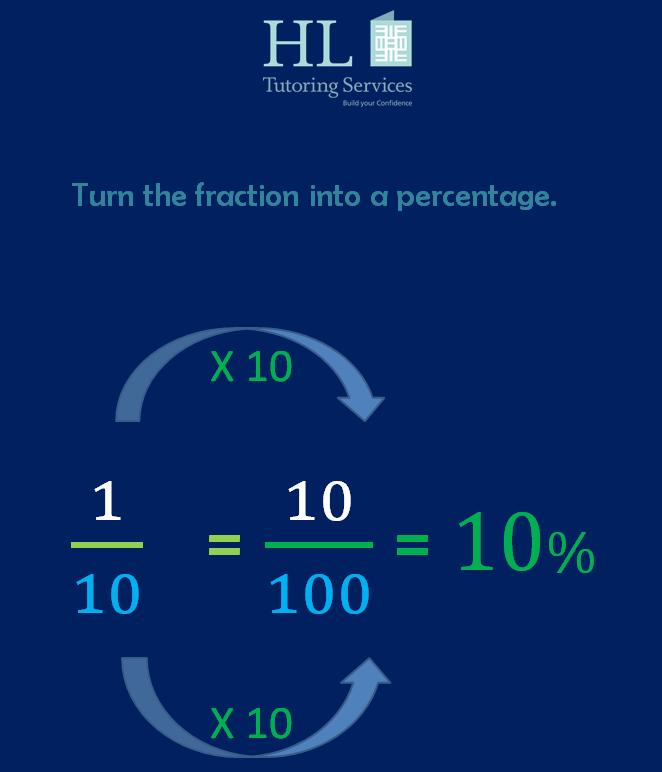top of pageSearch

Turn the percentage into a fraction so that you are working with the same type of numbers.

Find a common denominator for the fractions.

Fortieths are chosen as 4, 5 and 8 are common factors of 40. These numbers all 'go into' 40.

40 ÷ 4 = 10 or 10 X 4 = 40

40 ÷ 5 = 8 or 8 X 5 = 40

40 ÷ 8 = 5 or 5 X 8 = 40

Therefore quarters, fifths and eighths can be converted into fortieths.

To change two eighths into fortieths do the following:

Multiply the denominator by 5.

8 X 5 = 40

Multiply the numerator by 5, the same number.

2 X 5 = 10

So the number of fortieths required is 10.

Two eighths = ten fortieths

To work out the percentage of the total, add up all the fortieths and then subtract the total number of fortieths from a whole.

Convert the fortieths into a percentage. To do this, change the fortieths into tenths first.

Change the tenths into hundredths. Then change the hundredths into a percentage.Calculate a tenth of a million dollars which is also equivalent to ten percent of a million dollars.

Did you solve the problem another way? Please share your method and answers with HL Tutoring Services.

If you're interested in getting maths tuition then check the HL Tutoring Services website for further information. Alternatively you can book private lessons online by selecting either:

*Pay weekly

*Pay monthly

*Pay termly

After that, choose a suitable date and time.